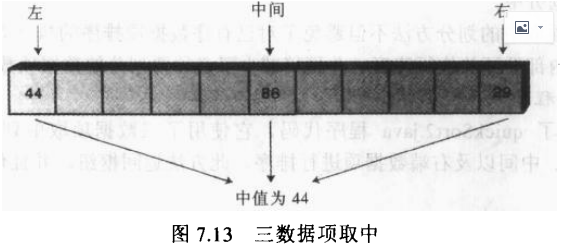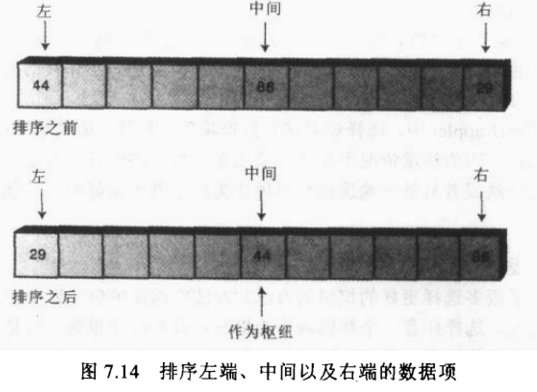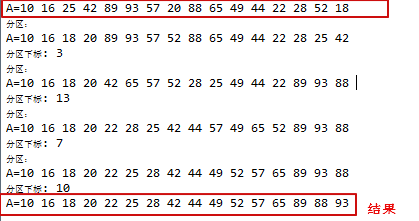# 快速排序算法(优化)

"三数据项取中"划分不同处在于枢纽选择做了优化

package com.vincent.suanfa;

public class quickSort1 {

public static void main(String[] args){
int maxSize = 16;
ArrayIns arr;
arr = new ArrayIns(maxSize);
//填充数组
for(int j=0; j < maxSize; j++){
long n = (int)(java.lang.Math.random()*99); //生成随机数
arr.insert(n);
}
arr.display();
arr.quickSort();
arr.display();
}
}

class ArrayIns{
private long[] theArray; //数组
private int nElems; //标识数组最大下标(即长度-1)

//构造函数
public ArrayIns(int max){
theArray = new long[max];
nElems = 0;
}

//把数据插入数组
public void insert(long value){
theArray[nElems] = value;
nElems++;
}

//打印数组
public void display(){
System.out.print("A=");
for(int j=0; j<nElems; j++){
System.out.print(theArray[j] + " ");
}
System.out.println("");
}

public void quickSort(){
recQuickSort(0,nElems-1);
}

public void recQuickSort(int left, int right){

int size = right - left +1;
if(size<=3){
manualSort(left,right);
}else{
long median = medianOf3(left,right);
int partition = partitionIt(left, right, median);
recQuickSort(left, partition-1);
recQuickSort(partition+1, right);
}
}

public long medianOf3(int left,int right){
int center = (left+right)/2;

if(theArray[left] > theArray[center])
swap(left,center);
if(theArray[left] > theArray[right])
swap(left,right);
if(theArray[center]>theArray[right])
swap(center,right);

swap(center,right-1);  //put pivot on right 把枢纽放到右边
return theArray[right-1];  //返回中间值
}

//分区函数
public int partitionIt(int left, int right, long pivot)
{
int leftPtr = left-1;
int rightPtr = right;
while(true)
{
//leftPtr表示数组下标为leftPtr时，其值小于pivot；目的是找到下标大于等于pivot的下标;即分出pivot左边的部分
while( theArray[++leftPtr] < pivot);

//rightPtr表示数组下标为rightPtr时，其值大于pivot;目的是找到下标小于等于pivot的下标;即分出pivot右边的部分
while(theArray[--rightPtr] > pivot);

if(leftPtr >= rightPtr)        //表示小于pivot的下标和大于pivot的下标交叉了，分区结束
break;
else
swap(leftPtr, rightPtr);  //把数据项按枢纽分成两组
}
swap(leftPtr, right);
System.out.println("分区：");
display();
System.out.println("分区下标: "+leftPtr);
return leftPtr;
}
//交换数据
public void swap(int dex1, int dex2)
{
long temp = theArray[dex1];
theArray[dex1] = theArray[dex2];
theArray[dex2] = temp;
}

public void manualSort(int left,int right){
int size = right - left + 1;
if(size<=1)
return;
if(size == 2)
{
if(theArray[left]>theArray[right])
swap(left,right);
return;
}else{
if(theArray[left] > theArray[right-1])
swap(left,right-1);
if(theArray[left] > theArray[right])
swap(left,right);
if(theArray[right-1]>theArray[right])
swap(right-1,right);
}
}
}posted @ 2020-05-05 10:42  Vincent-yuan  阅读(246)  评论(0编辑  收藏  举报